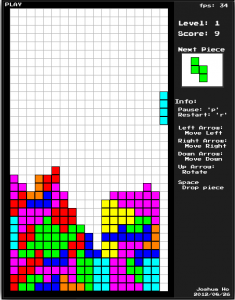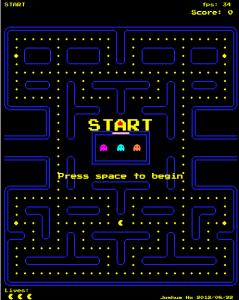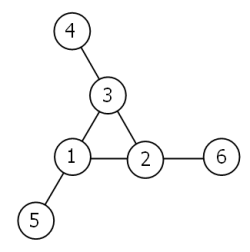### HTML5 – TetrisCompleted it in 2.5 days…
At this rate, I’m definitely going to need to step up my productivity for this if I want to make something comparable to Ludum Dare quality.
(2.5.. as a consolation it only included about 14 hours of coding.)

You can play it here.

### HTML5 – Pacman

I was talking to a colleague the other day about HTML5-Snake, and somehow the conversation drifted to Pacman…

After a weekend of investigating the Pacman (enemy) strategies, I took a break from doing EulerProject questions and began work on it.
It seemed simple enough: the background was pre-made, (sprites were handmade),  and it seemed very do-able.It took me 2 days for the base code, +1 for memory/cpu profiling.
It is without sound, but I think it’s quite playable.

You can try it out here.

### Project Euler – Problem 68

Problem 68:
Consider the following “magic” 3-gon ring, filled with the numbers 1 to 6, and each line adding to nine.### Project Euler – Problem 66

Problem 66:
Consider quadratic Diophantine equations of the form:

x^2 – D*y^2 = 1

### Project Euler – Problem 65

Problem 65:

The square root of 2 can be written as an infinite continued fraction.

sqrt(2) = 1+1/(2+(1/(2+1/(2+1/…))))

### Project Euler – Problem 64

Problem 64:
The first ten continued fraction representations of (irrational) square roots are:

### Project Euler – Problem 63

Problem 63:
The 5-digit number, 16807=7^5, is also a fifth power. Similarly, the 9-digit number, 134217728=8^9, is a ninth power.

How many n-digit positive integers exist which are also an nth power?

### Project Euler – Problem 62

Problem 62:
The cube, 41063625 (345^3), can be permuted to produce two other cubes: 56623104 (384^3) and 66430125 (405^3).

In fact, 41063625 is the smallest cube which has exactly three permutations of its digits which are also cube.

Find the smallest cube for which exactly five permutations of its digits are cube.

### Project Euler – Problem 61

Problem 61:
Triangle, square, pentagonal, hexagonal, heptagonal, and octagonal numbers are all figurate (polygonal) numbers and are generated by the following formulae:

```Triangle	 	P3,n=n(n+1)/2	 	1, 3, 6, 10, 15, ...
Square	 		P4,n=n2	 		1, 4, 9, 16, 25, ...
Pentagonal	 	P5,n=n(3n-1)/2	 	1, 5, 12, 22, 35, ...
Hexagonal	 	P6,n=n(2n-1)	 	1, 6, 15, 28, 45, ...
Heptagonal	 	P7,n=n(5n-3)/2	 	1, 7, 18, 34, 55, ...
Octagonal	 	P8,n=n(3n-2)	 	1, 8, 21, 40, 65, ...```

The ordered set of three 4-digit numbers: 8128, 2882, 8281, has three interesting properties.

The set is cyclic, in that the last two digits of each number is the first two digits of the next number (including the last number with the first).

### Project Euler – Problem 60

Problem 60:
The primes 3, 7, 109, and 673, are quite remarkable. By taking any two primes and concatenating them in any order the result will always be prime.